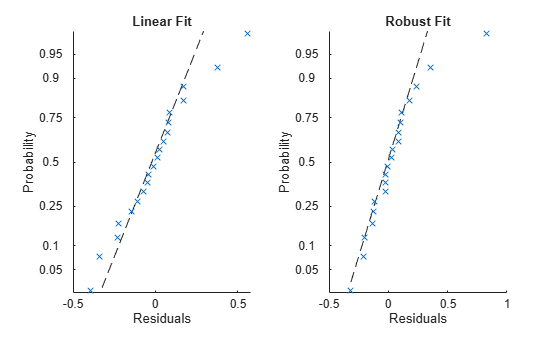## Robust Regression — Reduce Outlier Effects

### What Is Robust Regression?

The models described in What Is a Linear Regression Model? are based on certain assumptions, such as a normal distribution of errors in the observed responses. If the distribution of errors is asymmetric or prone to outliers, model assumptions are invalidated, and parameter estimates, confidence intervals, and other computed statistics become unreliable. Use `fitlm` with the `RobustOpts` name-value pair to create a model that is not much affected by outliers. The robust fitting method is less sensitive than ordinary least squares to large changes in small parts of the data.

Robust regression works by assigning a weight to each data point. Weighting is done automatically and iteratively using a process called iteratively reweighted least squares. In the first iteration, each point is assigned equal weight and model coefficients are estimated using ordinary least squares. At subsequent iterations, weights are recomputed so that points farther from model predictions in the previous iteration are given lower weight. Model coefficients are then recomputed using weighted least squares. The process continues until the values of the coefficient estimates converge within a specified tolerance.

### Robust Regression versus Standard Least-Squares Fit

This example shows how to use robust regression. It compares the results of a robust fit to a standard least-squares fit.

Step 1. Prepare data.

Load the `moore` data. The data is in the first five columns, and the response in the sixth.

```load moore X = [moore(:,1:5)]; y = moore(:,6);```

Step 2. Fit robust and nonrobust models.

Fit two linear models to the data, one using robust fitting, one not.

```mdl = fitlm(X,y); % not robust mdlr = fitlm(X,y,'RobustOpts','on');```

Step 3. Examine model residuals.

Examine the residuals of the two models.

```subplot(1,2,1) plotResiduals(mdl,'probability') subplot(1,2,2) plotResiduals(mdlr,'probability')```The residuals from the robust fit (right half of the plot) are nearly all closer to the straight line, except for the one obvious outlier.

Step 4. Remove the outlier from the standard model.

Find the index of the outlier. Examine the weight of the outlier in the robust fit.

```[~,outlier] = max(mdlr.Residuals.Raw); mdlr.Robust.Weights(outlier)```
```ans = 0.0246 ```

Check the median weight.

`median(mdlr.Robust.Weights)`
```ans = 0.9718 ```

This weight of the outlier in the robust fit is much less than a typical weight of an observation.